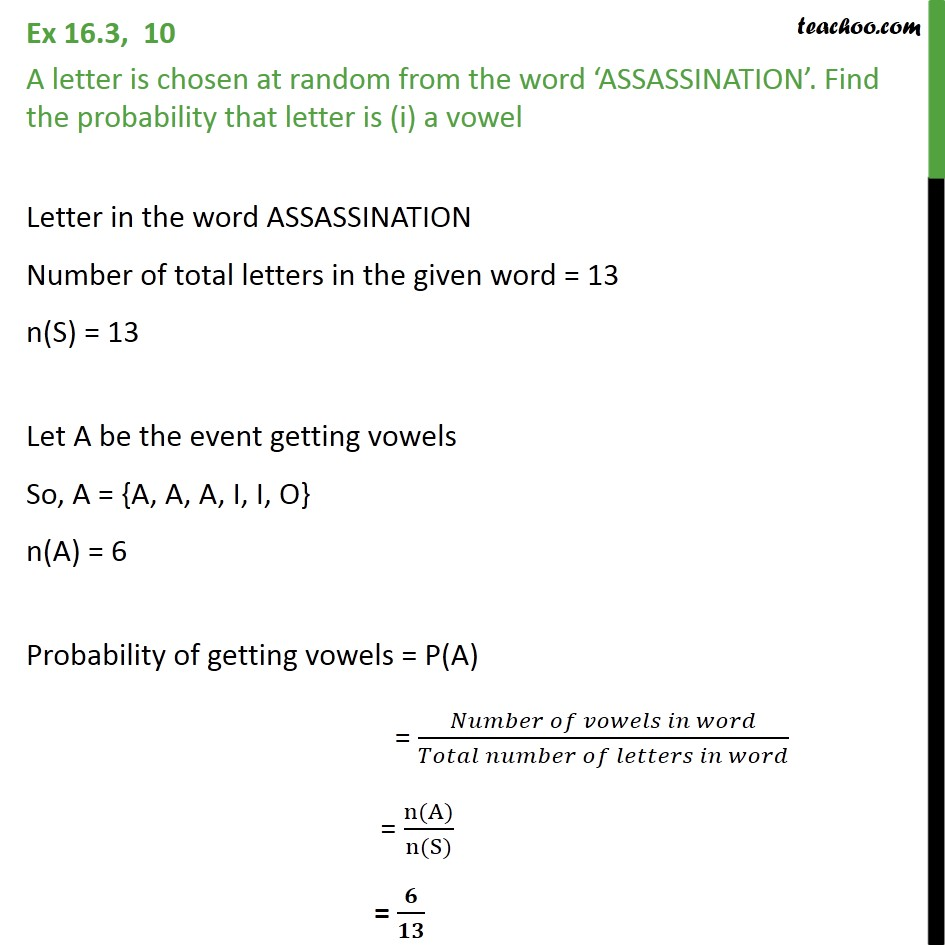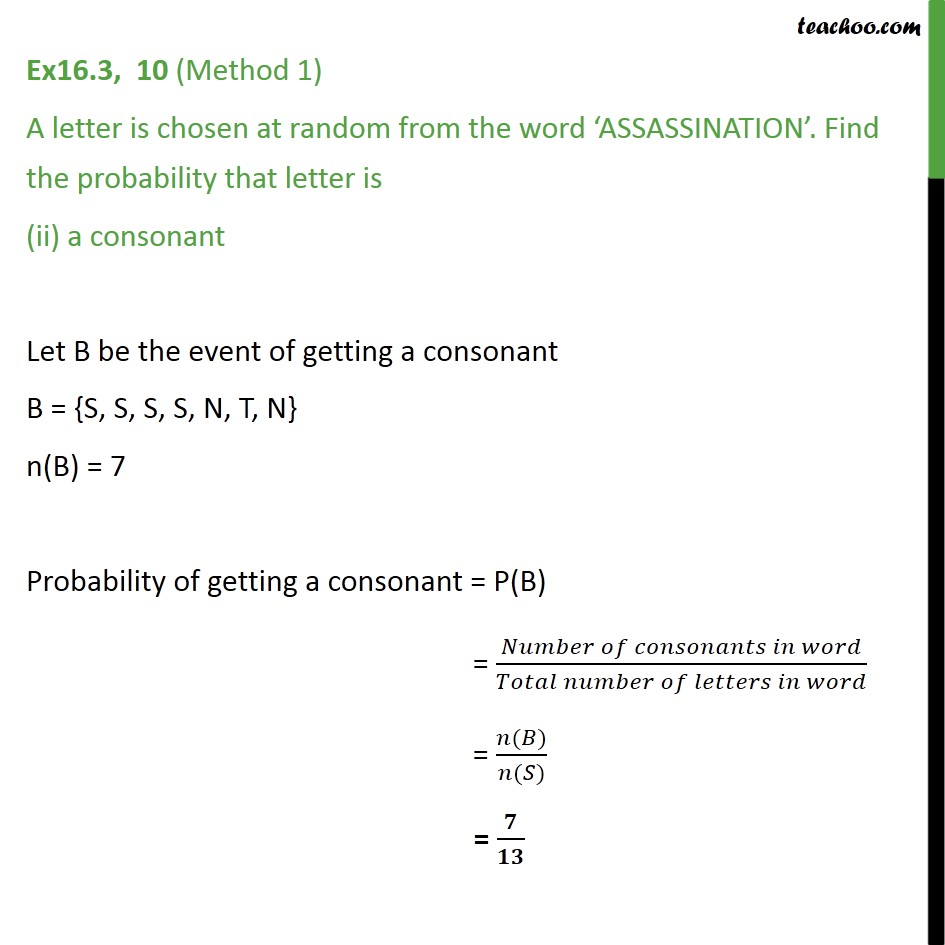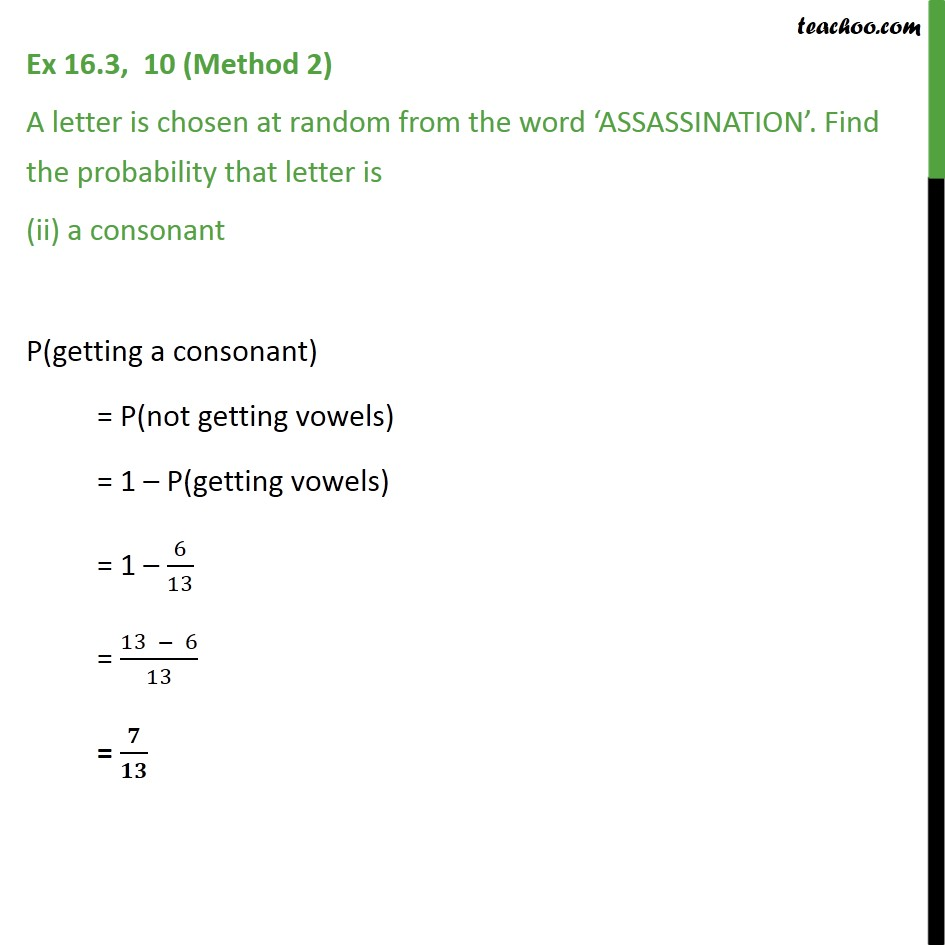Ex 14.2

Chapter 14 Class 11 Probability
Serial order wiseLearn in your speed, with individual attention - Teachoo Maths 1-on-1 Class

### Transcript

Ex 14.2, 10 A letter is chosen at random from the word ‘ASSASSINATION’. Find the probability that letter is (i) a vowel Letter in the word ASSASSINATION Number of total letters in the given word = 13 n(S) = 13 Let A be the event getting vowels So, A = {A, A, A, I, I, O} n(A) = 6 Probability of getting vowels = P(A) = 𝑁𝑢𝑚𝑏𝑒𝑟 𝑜𝑓 𝑣𝑜𝑤𝑒𝑙𝑠 𝑖𝑛 𝑤𝑜𝑟𝑑﷮𝑇𝑜𝑡𝑎𝑙 𝑛𝑢𝑚𝑏𝑒𝑟 𝑜𝑓 𝑙𝑒𝑡𝑡𝑒𝑟𝑠 𝑖𝑛 𝑤𝑜𝑟𝑑﷯ = n(A)﷮n(S)﷯ = 𝟔﷮𝟏𝟑﷯ Ex16.3, 10 (Method 1) A letter is chosen at random from the word ‘ASSASSINATION’. Find the probability that letter is (ii) a consonant Let B be the event of getting a consonant B = {S, S, S, S, N, T, N} n(B) = 7 Probability of getting a consonant = P(B) = 𝑁𝑢𝑚𝑏𝑒𝑟 𝑜𝑓 𝑐𝑜𝑛𝑠𝑜𝑛𝑎𝑛𝑡𝑠 𝑖𝑛 𝑤𝑜𝑟𝑑﷮𝑇𝑜𝑡𝑎𝑙 𝑛𝑢𝑚𝑏𝑒𝑟 𝑜𝑓 𝑙𝑒𝑡𝑡𝑒𝑟𝑠 𝑖𝑛 𝑤𝑜𝑟𝑑﷯ = 𝑛(𝐵)﷮𝑛(𝑆)﷯ = 𝟕﷮𝟏𝟑﷯ Ex 14.2, 10 (Method 2) A letter is chosen at random from the word ‘ASSASSINATION’. Find the probability that letter is (ii) a consonant P(getting a consonant) = P(not getting vowels) = 1 – P(getting vowels) = 1 – 6﷮13﷯ = 13 − 6﷮13﷯ = 𝟕﷮𝟏𝟑﷯